Mathematical and Physical Journal
for High Schools
Issued by the MATFUND Foundation
 Already signed up? New to KöMaL?

#Problem I. 331. (November 2013)

I. 331. Ancient Egyptians expressed (almost all) fractions as a sum of simple fractions with unit numerator. For example, 3/8 was given as 1/4+1/8 on Egyptian papyruses; they did not use the trivial but longer 1/8+1/8+1/8 form. In addition, they tried to express every operation with the help of addition and subtraction. Instead of multiplication and division, they used doubling and halving most of the time. As an example, let us consider how they computed 13 multiplied by 17''.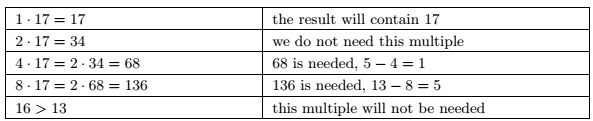The right column should be read from bottom to top, so the result is 136+68+17=221.

Performing the division of two integers was not a simple task either, as our next example illustrates. According to their papyruses, they computed 62 divided by 13'' as follows.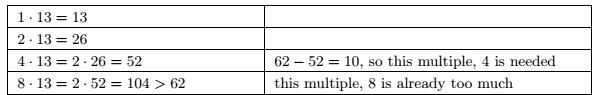Now they had to compute 10 divided by 13'', which was performed by repeated halving. The right column in the next table should be read from top to bottom.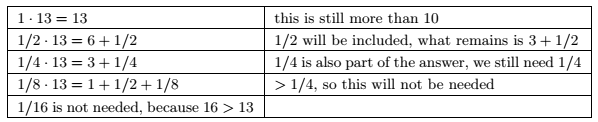The last step is to answer that 1/4 is which number multiplied by 13''. So they considered the successive halves of 1/13: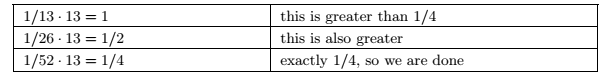The result of 62/13 is therefore 4+1/2+1/4+1/52. Since the numerator of each fraction is 1, this last form can be shortened and they simply wrote 4 /2 /4 /52.

You can find more information on Egyptian fractions and Egyptian algorithms on the Internet; in Hungarian, for example, in the book of Márton Sain (Nincs királyi út) from the Hungarian Electronic Library.

Your program i331 should perform multiplication or division of two positive integers below one million by using the Egyptian algorithm and format described above. Your program should process each line of the input file (given as the first command line argument to your program), and write the results (one result per line) into a file specified by the second command line argument. In the last table, Példa bemenet'' is a sample input, and Példa kimenet'' is the corresponding output.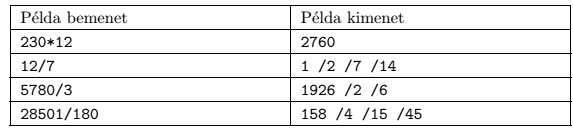The source code (i331.pas, i331.cpp, ...) together with a short documentation (i331.txt, i331.pdf, ...) - also describing which developer environment to use for compiling your source - should be submitted in a compressed file i331.zip.

(10 pont)

Deadline expired on December 10, 2013.

Sorry, the solution is available only in Hungarian. Google translation

Mintamegoldásként Csernák Tamás budapest, 12. évfolyamos diák Pascal nyelven készült (i331.pas) és Kovács Balázs Marcell budapest, 11. osztályos versenyző C++ nyelven írt (i331.cpp) munkáját adjuk közre.

### Statistics:

 8 students sent a solution. 10 points: Csernák Tamás, Kovács Balázs Marcell. 8 points: 1 student. 6 points: 1 student. 5 points: 2 students. 4 points: 1 student. 0 point: 1 student.

Problems in Information Technology of KöMaL, November 2013